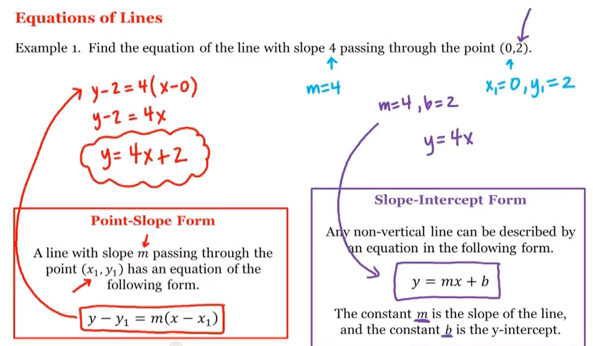# Home

## Math 1A/1B. Pre-Calculus: Equations of Lines (English)

UCI Math 1A/1B: Pre-Calculus
Pre-Calculus: Equation of Lines
View the complete course: http://ocw.uci.edu/courses/math_1a1b_precalculus.html
Instructor: Sarah Eichhorn, Ph.D and Rachel Lehman, Ph.D

More courses at http://ocw.uci.edu

Description: UCI Math 1A/1B: Precalculus is designed to prepare students for a calculus course. This course is taught so that students will acquire a solid foundation in algebra and trigonometry. The course concentrates on the various functions that are important to the study of the calculus.Author:
Sarah Eichhorn
Title:
Lecturer
Department:
Mathematics
Author:
Rachel Lehman
Title:
Lecturer
Department:
Mathematics